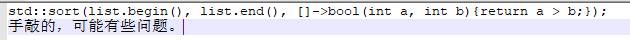2020-05-26 04:22

# c++ sort排序二维数组之后结果未自动保存的问题

5

LeetCode 1128,

https://leetcode.com/problems/number-of-equivalent-domino-pairs/

``````class Solution {
public:
int numEquivDominoPairs(vector<vector<int>>& dominoes) {
int ret = 0;
for (auto i : dominoes) {
sort(i.begin(), i.end());
cout << i << i << endl;   // 输出1
}
for (int i = 0; i < dominoes.size() - 1; ++i) {
for (int j = i + 1; j < dominoes.size(); ++j) {
cout << dominoes[i] << dominoes[i] << endl;  // 输出2
cout << dominoes[j] << dominoes[j] << endl;
if (dominoes[i] == dominoes[j]) {
ret++;
}
}
}
return ret;
}
};

int main() {
vector<vector<int>> vec = { {1,2}, {2,1} };
Solution s;
cout << s.numEquivDominoPairs(vec) << endl;
return 0;
}

``````

• 点赞
• 写回答
• 关注问题
• 收藏
• 复制链接分享
• 邀请回答

#### 2条回答

• ``````for (auto i : dominoes)
->
for (auto& i : dominoes)
``````
点赞 1 评论 复制链接分享
•点赞 评论 复制链接分享